×#### Thank you for registering.

One of our academic counsellors will contact you within 1 working day.

Click to Chat

1800-1023-196

+91-120-4616500

CART 0

• 0

MY CART (5)

Use Coupon: CART20 and get 20% off on all online Study Material

ITEM
DETAILS
MRP
DISCOUNT
FINAL PRICE
Total Price: Rs.

There are no items in this cart.
Continue Shopping• Complete Physics Course - Class 11
• OFFERED PRICE: Rs. 2,968
• View Details

Maximum Power Transfer Theorem

Table of Content

Alternating Current

Power in an AC Circuit

Choke coil

Construction

Related Resources

Alternating Current

As we have seen earlier a rotating coil in a magnetic field, induces an alternating emf and hence an alternating current. Since the emf induced in the coil varies in magnitude and direction periodically, it is called an alternating emf. The significance of an alternating emf is that it can be changed to lower or higher voltages conveniently and efficiently using a transformer. Also the frequency of the induced emf can be altered by changing the speed of the coil. This enables us to utilize the whole range of electromagnetic spectrum for one purpose or the other. For Example domestic power in India is supplied at a frequency of 50 Hz. For transmission of audio and video signals, the required frequency range of radio waves is between 100 KHz and 100 MHz. Thus owing to its wide applicability most of the countries in the world use alternating current.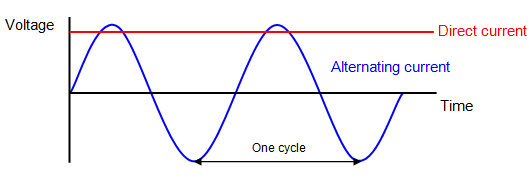Power in an AC Circuit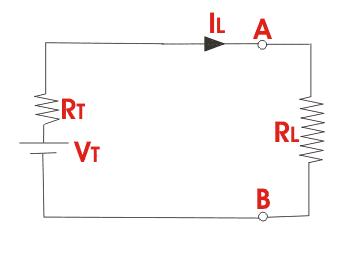In an a.c circuit the current and emf vary continuously with time. Therefore power at a given instant of time is calculated and then its mean is taken over a complete cycle. Thus, we define instantaneous power of an a.c. circuit as the product of the instantaneous emf and the instantaneous current flowing through it.

The instantaneous value of emf and current is given by

e = Eo sin ωt

i = Io sin (ωt + φ)

where φ is the phase difference between the emf and current in an a.c circuit.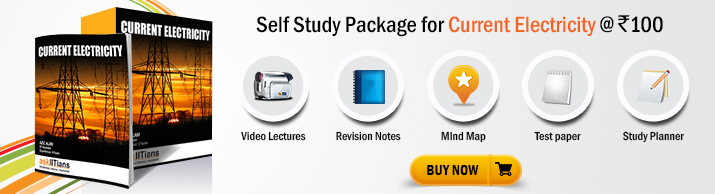The average power consumed over one complete cycle is

On simplification, we obtain

Pav = (E0I0/2) cosφ

Pav = (E0/√2) (I0/√2)cosφ = Erms Irms cosφ

Pav = apparent power  power factor

where Apparent power = Erms Irms and power factor = cos φ

The average power of an ac circuit is also called the True Power of the Circuit.

Refer this video to know more about on maximum power transfer theorem

Choke coil

A choke coil is an inductance coil of very small resistance used for controlling current in an a.c. circuit. If a resistance is used to control current, there is wastage of power due to Joule heating effect in the resistance. On the other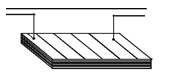hand there is no dissipation of power when a current flows through a pure inductor.

Construction

It consists of a large number of turns of insulated copper wire wound over a soft iron core. A laminated core is used to minimise eddy current loss as shown in figure.

Working

The inductive reactance offered by the coil is given by

XL = ωL

In the case of an ideal inductor the current lags behind the emf

by a phase angle π/2 .

Thus,the average power consumed by the choke coil over a complete cycle is

Pav = Erms Irms cos π/2 = 0

However in practice, a choke coil of inductance L possesses a small resistance r. Hence it may be treated as a series combination of an inductor and small resistance r. In that case the average power consumed by the choke coil over a complete cycle is

Pav = ErmsIrmscos φ

Pav = ErmsIrms [r/(√r2+ω2L2)]        …... (1)

Here [r/(√r2+ω2L2)]  is the power factor. From equation (1), the value of average power dissipated works out to be much smaller than the power loss I2R in a resistance R.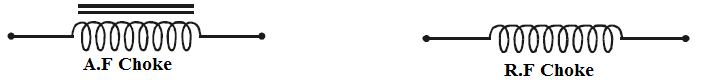Chokes used in low frequency a.c. circuit have an iron core so that the inductance may be high. These chokes are known as audio – frequency (A.F) chokes. For radio frequencies, air chokes are used since a low inductance is sufficient. These are called Radio Frequency (R. F) or High Frequency (H.F) chokes and are used in wireless receiver circuits (Fig. 4.24a and Fig. 4.24b).

Choke coils can be commonly seen in fluorescent tubes which work on alternating currents.Increase in R, decreases φ, thereby, increasing cosφ. This results in an increase in actual power consumed.

An increase in L and/or C results in an increase in φ, thus, decreasing cosφ. This tends to decrease the actual power consumed in the circuit.

The maximum amount of power will be dissipated by a load resistance when that load resistance is equal to the Thevenin/Norton resistance of the network supplying the power.

If the load resistance is lower or higher than the Thevenin/Norton resistance of the source network, its dissipated power will be less than maximum.

Maximum power transfer theoremdetermines the value of resistance RL for which, the maximum power will be transferred from source to it.

The maximum power, drawn from the source, depends upon the value of the load resistance.

The maximum power transfer theorem defines the condition under which the maximum power is transferred to the load in a circuit.

The maximum power transfer theorem is sometimes called the conjugate match thoerem.

A resistance is connected to a capacitor in AC and the phase difference is π/4 between current and voltage. When the same resistance is connected to an inductor, phase difference becomes tan-1(2). Power factor of the circuit when both capacitor and inductor are connected to the resistance will be,

(a) 1

(b) 1/√2

(c) 1/√3

(d) ½

Solution:

We know that, tan π/4 = XL/R

So, XL = R

tan-1 (2) = XC/R

Or, XC/R = 2

So, XC = 2R

Now, in L-C-R circuit

Z = √[R2+(XC – XL)2] =√2R

Thus, power factor = cos θ = R/Z = 1/√2

Therefore, from the above observation we conclude that, option (b) is correct.

Problem:

A copper wire having a cross-sectional area of 0.5 mm2 and length of 0.1 m is initially at 25oC and is thermally insulated from the surroundings. If a current of 10 A is set up in this wire,

(a) find the time in which the wire starts melting. [The change of resistance of the wire with temperature may be neglected.]

(b) What will this time be, if the length of the wire is doubled?

[Density of Cu = 9 × 103 kg m–3, specific heat of Cu = 9 × 10–2 cal kg–1 oC–1, M.P. (Cu) = 1075 oC and specific resistance =

1.6 × 10–8 Wm].

Solution:

(a) Mass of Cu = Volume × density = 0.5 × 10–6 × 0.1 × 9 ×

103 = 45 × 10–5 kg.

Rise in temperature = q = 1075 – 25 = 1050 oC. Specific

heat = 9 × 10–2 kg–1 oC × 4.2 J

Þ l2Rt = mSq

Þ t = mSq/l2.R

But R = pL / A = 1.6 × 10–8 × 0.1 / 0.5 × 10–8 = 0.558 s

(b) When the length of wire is doubled, R is doubled, but correspondingly mass is also doubled. Therefore, the wire will start melting in the same time.Question 1

The alternating current is that which:

(a) flows intermittently

(b) varies in magnitude

(c) gets only its direction reserved after equal intervals of time

(d) changes continuously in magnitude and reverses periodically in direction.

Question 2

Power factor of an a.c. circuit is:

(a) always greater than one

(b) always less than one

(c) always equal to one

(d) can have any value

Question 3

A choke coil is used as a resistance in:

(a) a.c. circuit

(b) d.c. circuit

(c) d.c. potentiometer circuit

(d) Wheatstone circuit

Question 4

A choke coil is a coil with:

(a) low inductance and high resistance

(b) low inductance and low resistance

(c) high inductance and high resistance

(d) high inductance and negligibly small resistance

Question 5

In purely inductive circuits, the current:

(a) Lags behind voltage by π/2

(b) Leads the voltage by π/2

(c) Is in phase with the voltage

(d) None of the aboveQ.1
Q.2
Q.3
Q.4
Q.5

d

b

a

d

a

Related Resources

You might like to drift velocity and Ohm’s law.

For getting an idea of the type of questions asked, refer the  Previous Year Question Papers.

To read more, Buy study materials of Current Electricity comprising study notes, revision notes, video lectures, previous year solved questions etc. Also browse for more study materials on Physics here.### Course Features

• 101 Video Lectures
• Revision Notes
• Previous Year Papers
• Mind Map
• Study Planner
• NCERT Solutions
• Discussion Forum
• Test paper with Video Solution